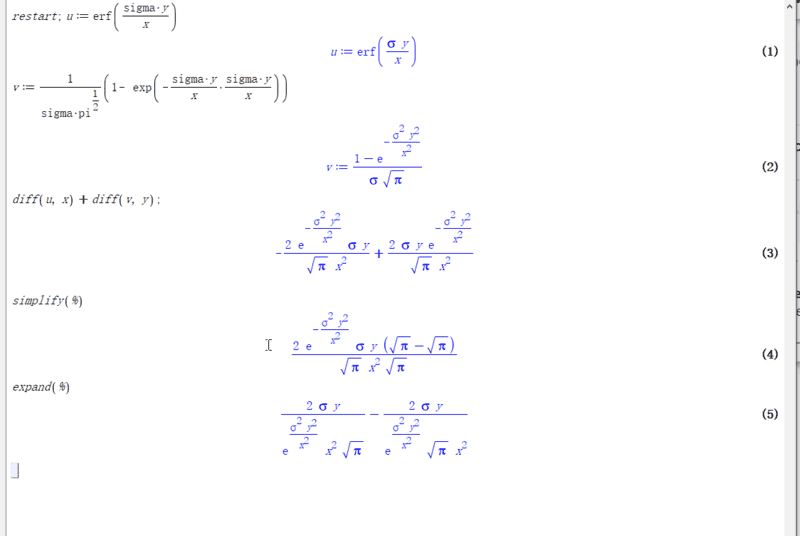# Why can't Maple simplify this further?

• Maple
• ztdep

#### ztdep

I am evaluating this formula, it's real value is 0. but maple can't further simplify it?Probably because (√π - √π ) isn't necessarily zero. It depends which of the two square roots you take. If you force it to take the positive square roots, then it will be zero.

••SolarisOne, PeroK, Wrichik Basu and 1 other person
How can (√π - √π) not be zero? We are not solving a quadratic equation here, so ##\sqrt{\pi} > 0##.

How can (√π - √π) not be zero?

If you select a branch at random each time you write a $\sqrt{}$.

It may be that Maple thinks that each $\pi$ is a separate variable, whose values are not necessarily equal.

Probably because (√π - √π ) isn't necessarily zero. It depends which of the two square roots you take.

How can (√π - √π) not be zero? We are not solving a quadratic equation here, so ##\sqrt π>0##.
To elaborate on @DrClaude's comment, the square root of a positive real number is by convention the principal square root of that number. i.e., the positive square root.

Although the equation ##x^2 - 4 = 0## has two solutions -- x = 2 or x = -2, it is an error to say that ##\sqrt 4 = \pm 2##.

•PeroK
I am evaluating this formula, it's real value is 0. but maple can't further simplify it?
Try entering Pi instead of pi.
For Maple, the first one is the famous mathematical constant, the second one is an undefined variable.

••I like Serena, PeroK and berkeman
To elaborate on @DrClaude's comment, the square root of a positive real number is by convention the principal square root of that number. i.e., the positive square root.

Although the equation ##x^2 - 4 = 0## has two solutions -- x = 2 or x = -2, it is an error to say that ##\sqrt 4 = \pm 2##.
Conventions are one thing, but what's coded up in the Maple code is another. What's your explanation for why it didn't replace (√π - √π ) in equation 4 of the OP with 0?

Conventions are one thing, but what's coded up in the Maple code is another.
##\sqrt \pi## in Maple must be a positive number. I'd be very surprised if something as basic as that was coded wrongly.

Maybe try to actually run it through Maple with reference to post #6.
It is a triviality, nothing else. And it certainly does not have anything to do with branches of square roots.

Conventions are one thing, but what's coded up in the Maple code is another. What's your explanation for why it didn't replace (√π - √π ) in equation 4 of the OP with 0?
No idea, but I'm also puzzled why Maple added two expressions whose denominators were both ##\sqrt \pi x^2## to get a single expression with a denominator of ##\sqrt \pi x^2 \sqrt \pi##.

However, I think @S.G. Janssens has hit the nail on the head with his advice to use Pi rather than pi. The Maple documentation backs up this advice - https://www.maplesoft.com/support/help/maple/view.aspx?path=initialconstants.